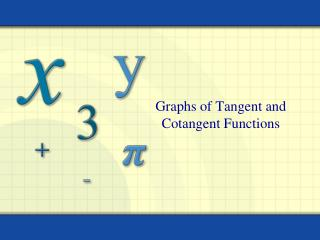Download PresentationGraphs of Tangent and Cotangent Functions

Graphs of Tangent and Cotangent Functions - PowerPoint PPT Presentation

Graphs of Tangent and Cotangent Functions. Plan for the Day. Review Homework Graphing Tangent and Cotangent Homework. Key Steps in Graphing Sine and Cosine. Identify the key points of your basic graph Find the new period (2 π / b ) Find the new beginning ( bx - c = 0)I am the owner, or an agent authorized to act on behalf of the owner, of the copyrighted work described.
Download PresentationGraphs of Tangent and Cotangent Functions

Download Policy: Content on the Website is provided to you AS IS for your information and personal use and may not be sold / licensed / shared on other websites without getting consent from its author.While downloading, if for some reason you are not able to download a presentation, the publisher may have deleted the file from their server.

- - - - - - - - - - - - - - - - - - - - - - - - - - E N D - - - - - - - - - - - - - - - - - - - - - - - - - -
Presentation Transcript
1. Graphs of Tangent and Cotangent Functions

2. Plan for the Day • Review Homework • Graphing Tangent and Cotangent • Homework

3. Key Steps in Graphing Sine and Cosine Identify the key points of your basic graph • Find the new period (2π/b) • Find the new beginning (bx - c = 0) • Find the new end (bx - c = 2π) • Find the new interval (new period / 4) to divide the new reference period into 4 equal parts to create new x values for the key points • Adjust the y values of the key points by applying the amplitude (a) and the vertical shift (d) • Graph key points and connect the dots

4. Key Steps in Graphing Secant and Cosecant • Identify the key points of your reciprocal graph (sine/cosine), note the original zeros, maximums and minimums • Find the new period (2π/b) • Find the new beginning (bx - c = 0) • Find the new end (bx - c = 2π) • Find the new interval (new period / 4) to divide the new reference period into 4 equal parts to create new x values for the key points • Adjust the y values of the key points by applying the amplitude (a) and the vertical shift (d) • Using the original zeros, draw asymptotes, maximums become minimums, minimums become maximums… • Graph key points and connect the dots based upon known shape

5. Tangent and Cotangent Look at: • Shape • Key points • Key features • Transformations

6. Graph Set window Domain: -2π to 2π x-intervals: π/2 (leave y range) Graph y = tan x

7. To graph y = tan x, use the identity . y Properties of y = tan x 1. domain : all real x x 4. vertical asymptotes: period: Graph of the Tangent Function At values of x for which cos x = 0, the tangent function is undefined and its graph has vertical asymptotes. 2. range: (–, +) 3. period: 

8. Graph y = tan x and y = 4tan x in the same window What do you notice? y = tan x and y = tan 2x What do you notice? y = tan x and y = -tan x What do you notice?

9. Graph Set window Domain: 0 to 2π x-intervals: π/2 (leave y range) Graph y = cot x

10. y To graph y = cot x, use the identity . Properties of y = cot x x 1. domain : all real x 4. vertical asymptotes: vertical asymptotes Graph of the Cotangent Function Cotangent Function At values of x for which sin x = 0, the cotangent function is undefined and its graph has vertical asymptotes. 2. range: (–, +) 3. period: 

11. Graph Cotangent y = cot x and y = 4cot x in the same window What do you notice? y = cot x and y = cot 2x What do you notice? y = cot x and y = -cot x What do you notice? y= cot x and y = -tan x

12. Key Steps in Graphing Tangent and Cotangent Identify the key points of your basic graph • Find the new period (π/b) • Find the new beginning (bx - c = 0) • Find the new end (bx - c =π) • Find the new interval (new period / 2) to divide the new reference period into 2 equal parts to create new x values for the key points • Adjust the y values of the key points by applying the amplitude (a) and the vertical shift (d) • Graph key points and connect the dots

13. Homework 27 • Page 318 • 1-6 all (matching), sketch the graph 19, 24